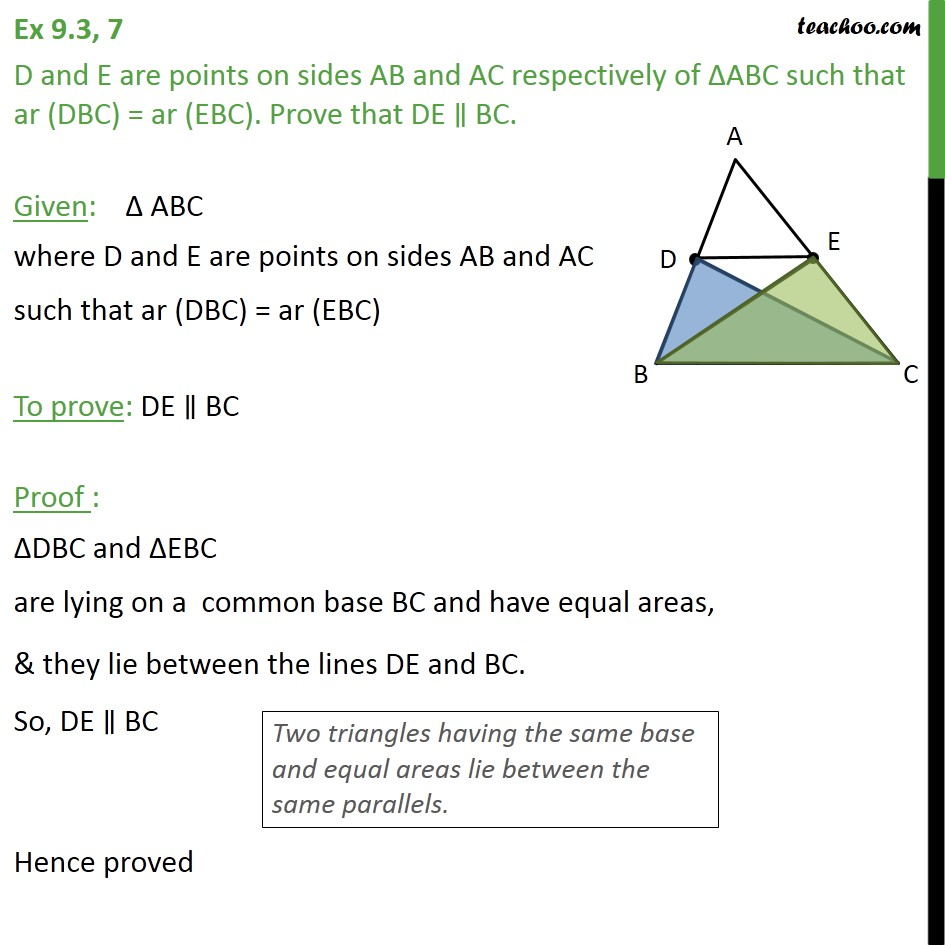Area of Triangles

Areas of Parallelograms and Triangles
Serial order wiseLearn in your speed, with individual attention - Teachoo Maths 1-on-1 Class

### Transcript

Question 7 D and E are points on sides AB and AC respectively of ABC such that ar (DBC) = ar (EBC). Prove that DE BC. Given: ABC where D and E are points on sides AB and AC such that ar (DBC) = ar (EBC) To prove: DE BC Proof : DBC and EBC are lying on a common base BC and have equal areas, & they lie between the lines DE and BC. So, DE BC Hence proved